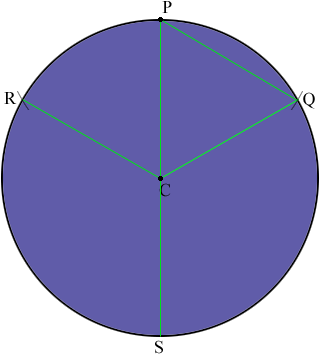SEARCH HOMEMath Central Quandaries & QueriesQuestion from Brian: We have to line up 3 pins on a lifting bridle to be 120 degrees apart from each other to connect into a lifting assembly. I know pi*d. I know straight lines connecting these points to each other will result in an equilateral triangle. How does one find these points without using a protractor? There must be a formula (formulae) to work this out mathematically. r = 10.5 inches pi*d = 65.9736 3 segments( arcs) = 21.9912 which is the distance along the circumference that the points are from each other.Hi Brian,

Is a compass allowed? If so draw a circle of radius 10.5 inches with center C, and mark a point P on the circumference. With the compass setting unchanged put the point at P and mark two small arcs to intersect the circle at Q and R.Since the line segments PC, CQ and QP are of equal length the triangle PCQ is equilateral and hence the measure of the angle PCQ is 60 degrees. In a similar fashion the measure of the angle RCP is 60 degrees and thus the measure of the angle RCQ is 120 degrees.

Finally extend the line PC to meet the circle at S. Q, R and S are the three points you need.

PennyMath Central is supported by the University of Regina and The Pacific Institute for the Mathematical Sciences.